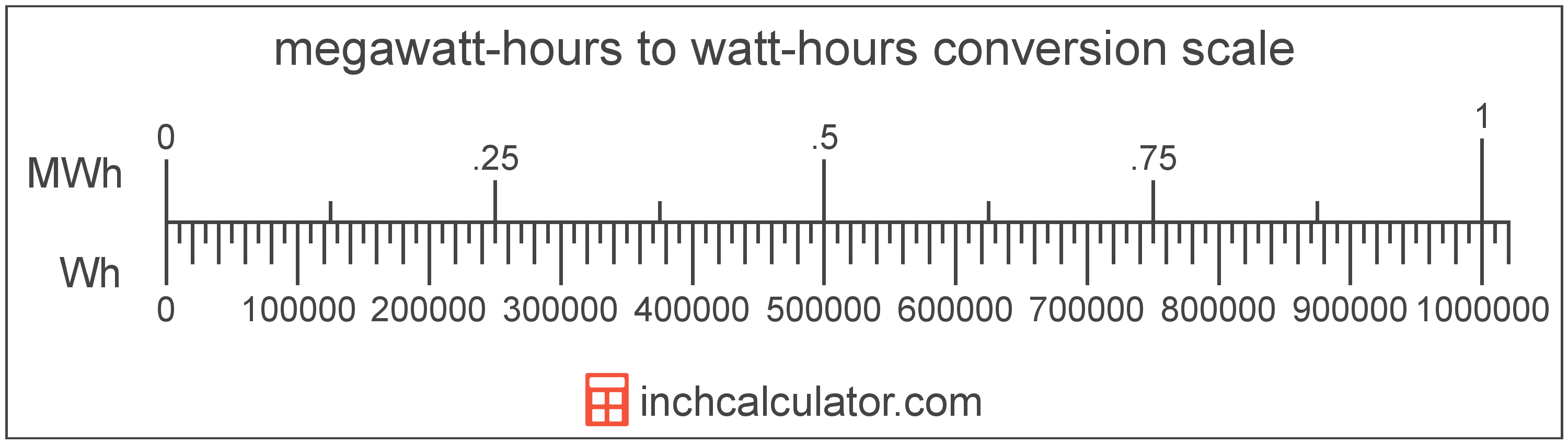# Watt-hours to Megawatt-hours Conversion

Enter the energy in watt-hours below to get the value converted to megawatt-hours.

Results in Megawatt-hours:1 Wh = 1.0E-6 MWh
Hint: use a scientific notation calculator to convert E notation to decimal

## How to Convert Watt-hours to Megawatt-hoursTo convert a watt-hour measurement to a megawatt-hour measurement, divide the energy by the conversion ratio.

Since one megawatt-hour is equal to 1,000,000 watt-hours, you can use this simple formula to convert:

megawatt-hours = watt-hours ÷ 1,000,000

The energy in megawatt-hours is equal to the watt-hours divided by 1,000,000.

For example, here's how to convert 500,000 watt-hours to megawatt-hours using the formula above.
500,000 Wh = (500,000 ÷ 1,000,000) = 0.5 MWh

## Watt-hours

The watt-hour is a measure of electrical energy equal to one watt of power over a one hour period.

Watt-hours are usually abbreviated as Wh, although the formally adopted expression is W·h. The abbreviation W h is also sometimes used. For example, 1 watt-hour can be written as 1 Wh, 1 W·h, or 1 W h.

In formal expressions, the centered dot (·) or space is used to separate units used to indicate multiplication in an expression and to avoid conflicting prefixes being misinterpreted as a unit symbol.

## Megawatt-hours

A megawatt-hour is a measure of electrical energy equal to one megawatt, or 1,000,000 watts, of power over a one hour period. Megawatt-hours are a measure of electrical work performed over a period of time, and are often used as a way of measuring energy usage by electric companies.

Megawatt-hours are usually abbreviated as MWh, although the formally adopted expression is MW·h. The abbreviation MW h is also sometimes used. For example, 1 megawatt-hour can be written as 1 MWh, 1 MW·h, or 1 MW h.

## Watt-hour to Megawatt-hour Conversion Table

Watt-hour measurements converted to megawatt-hours
Watt-hours Megawatt-hours
1 Wh 0.000001 MWh
2 Wh 0.000002 MWh
3 Wh 0.000003 MWh
4 Wh 0.000004 MWh
5 Wh 0.000005 MWh
6 Wh 0.000006 MWh
7 Wh 0.000007 MWh
8 Wh 0.000008 MWh
9 Wh 0.000009 MWh
10 Wh 0.00001 MWh
100 Wh 0.0001 MWh
1,000 Wh 0.001 MWh
10,000 Wh 0.01 MWh
100,000 Wh 0.1 MWh
1,000,000 Wh 1 MWh

## References

1. Bureau International des Poids et Mesures, The International System of Units (SI), 9th edition, 2019, https://www.bipm.org/documents/20126/41483022/SI-Brochure-9-EN.pdf